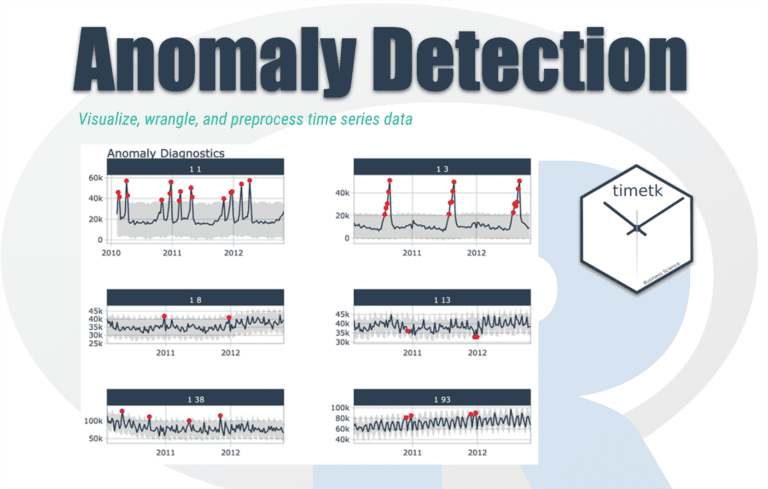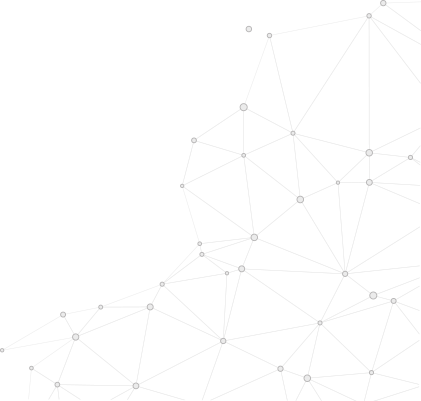## Anomaly Detection in a time series

Anomaly detection can be applied to a time series where we want to create a baseline model and determine the deviation of the observations with the baseline. From deviation, we can get information such that if it is large enough, the observation is deemed abnormal and is flagged. In general, novelty and outlier detection does not tell us why something is possibly an outlier, but the conditions and causes led to an unusual observation. For example, generally, there are cases where we observe server logs, anomalous observations may result from some equipment or code breakdown or something malignant like a security breach.

What should be your plan of attack be for analysing the time series?

So, generally, first, we visualise the data; after that, our goal is to generate the baseline model, so we can ask ourselves if there is any drift, and if present, we have to remove that from our data set. The other thing we need to ask ourselves is some seasonality or periodicity in our dataset. We have to find those and remove them from our model, so what’s left behind is the residual, we can do some further modelling on those residuals. So this is the overall plan of attack.

Fourier Analysis

While it is hard to tell, there are periodic behaviours in the time series. We can better spotlight the dominant frequencies that support the time series using Fourier analysis. So here, I have taken a Fourier analysis code to give an idea about the dominant frequencies.

Output Graph:

From this graph, we got to see that this time series has four dominant frequencies: daily, twice-daily, three times a day, and four times a day. In other words, 6, 8, 12, and 24 hour periods.

Initial baseline model

Generally, we create an initial baseline model to get an idea of the data and also to know whether the initial baseline model is adequate for the time series. Generally, before using the initial baseline model, we create some custom transformers (if needed) to work with our pandas time-series data. The most custom transformer is used to generate Fourier components, transform DateTime objects into a unit of time, etc. So after using the custom transformer and the initial baseline model, the residuals are generated to reveal whether the time series has a lot of shock events, a sudden increase in energy usage probably as a result of sudden and short use of products. So there’s a need to analyse the residuals for any temporal correlations.

Noice Based Features

The first thing we want to unveil is the correlation of past residuals with current values. An autocorrelation plot will inform us whether the time series elements are positively correlated, independent of each other or negatively correlated. In short, it tells the characteristic time scale of the process to guide us when generating noise-based features.

z-Score

Since there is little temporal correlation with residual values, we assume that the residuals are independently sampled from the same distribution. Given this probabilistic perspective, we can quantify the degree of an anomaly to each observation if we know the distribution the residuals are being sampled from. If the distribution has one peak, there is a lower probability of observing values far from the peak. The z-score is a relative measure of how far away a value is from the mean, normalised by the standard deviation.

How should we decide the appropriate z-score cutoff?

If we set the z-score large, so it will increase the range of normal points or inliers, and if we set the z-score small so it will decrease the range of normal points, so if we view there is no perfect answer for this, we should consider that it all depends on precision and recall we want in our analysis.

Rolling z-score

The calculation of the z-score relied on the entire time series for calculating the mean and standard deviation. We will usually be streaming observations for anomaly detection with time series, and the entire series will not be available. Instead, we can calculate the z-score on a window of observations rather than the whole time history. The advantage of rolling z-score is that not to hold a large amount of data in memory, and it also reflects the fact that it is better to use current values though the observation is anomalous and rolling z-score is more adaptive to recent changes in the process.### Get free consultation from our tech experts## Related articles you may would like to read

### FREE eBOOK: Recommended RFP process for selecting cloud provider

We are here to simply your migration to cloud by embarking on a systematic, planned, stage-wise journey to the cloud.

##### Categories
###### Resources